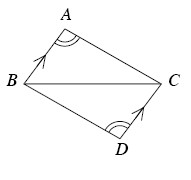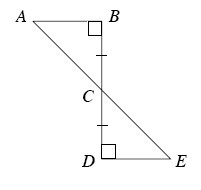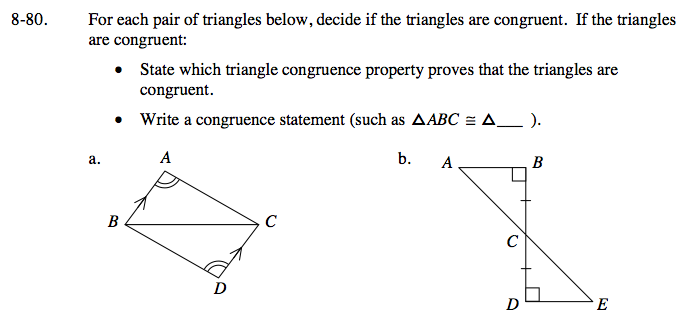Home > GC > Chapter 8 > Lesson 8.2.2 > Problem8-80

8-80.
1. For each pair of triangles below, decide if the triangles are congruent. If the triangles are congruent: Homework Help ✎

• State which triangle congruence property proves that the triangles are congruent.

• Write a congruence statement (such as ΔABC ≅ Δ ____).

1.2.What parts of these triangles makes them congruent?

AAS≅, ΔABC≅ΔDCB

Use the same method as part (a), but use a different congruence property.